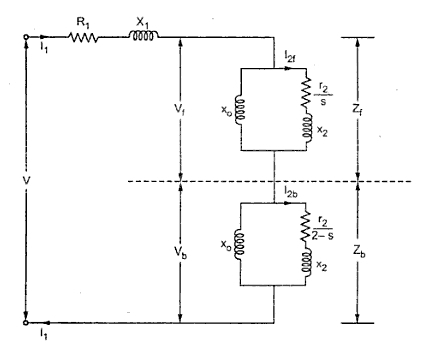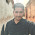### Equivalent Circuit of Single Phase Induction Motor

The double revolving field theory can be effectively used to obtain the equivalent circuit of a single phase induction motor. The method consists of determining the values of both the fields clockwise and anticlockwise at any given slip. When the two fields are known, the torque produced by each can be obtained. The difference between these two torques is the net torque acting on the rotor.
Imagine the single phase induction motor is made up of one stator winding and two imaginary rotor windings. One rotor is rotating in forward direction i.e. in the direction of rotating magnetic field with slip s while other is rotating in backward direction i.e. in direction of oppositely directed rotating magnetic field with slip 2 - s.
To develop the equivalent circuit, let us assume initially that the core loss is absent.
1. Without core loss
Let the stator impedance be Z Ω
Z = R1 + j X1
Where                  R1 = Stator resistance
X1 = Stator reactance
And                      X = rotor reactance referred to stator
R2 = rotor resistance referred to stator
Hence the impedance of each rotor is r2 + j x2   where
x2 = X2/2
The resistance of forward field rotor is r2/s while the resistance of backward field rotor is r2 /(2 - s). The r2 value is half of the actual rotor resistance referred to stator.
As the core loss is neglected, Ro is not existing in the equivalent circuit. The xo is half of the actual magnetising reactance of the motor. So the equivalent circuit referred to stator is shown in the Fig.1.Fig. 1  Equivalent circuit without core loss
Now the impedance of the forward field rotor is Zf  which is parallel combination of  (0 + j xo ) and (r2 /s) + j x2

While the impedance of the backward field rotor is Zb which is parallel combination of  (0 + j xo) and (r2 / 2-s) + j x2.
Under standstill condition, s = 1 and 2 - s = 1 hence Z= Zand hence Vf = Vb . But in the running condition, Vbecomes almost 90 to 95% of the applied voltage.
...                           Zeq  = Z + Z+ Z  = Equivalent impedance
Let                         I2f  = Current through forward rotor referred to stator
and                         I2b  = Current through backward rotor referred to stator
...                            I2f  = /((r2/s) + j x2) where V= I x Z
and                         I2b  = /((r2/2-s)  + j x2)
P= Power input to forward field rotor
= (I2f)2 (r2/s)  watts
Pb = Power input to backward field rotor
= (I2b)2 (r2/2-s) watts
P= (1 - s){ Net power input}
= (1 - s) (P- Pb ) watts
Pout = Pm - mechanical loss - core loss
...                           T = forward torque = Pf /(2πN/60) N-m
and                        Tb = backward torque = Pb /(2πN/60) N-m
T = net torque = T - T
while                       Tsh  = shaft torque = Pout /(2πN/60) N-m
%η = (net output / net input) x 100

2. With core loss
If the core loss is to be considered then it is necessary to connect a resistance in parallel with, in an exciting branch of each rotor is half the value of actual core loss resistance. Thus the equivalent circuit with core loss can be shown as in the Fig. 2.Fig. 2 Equivalent circuit with core loss

Let                    Zof  = Equivalent impedance of exciting branch in forward rotor
= ro║(j xo )
and                     Zob  = Equivalent impedance of exciting branch in backward rotor
= ro║(j xo )
...                          Z= Zof  ║( r2/s + j x2 )
All other expressions remains same as stated earlier in case of equivalent circuit without core loss.

1.can you tell us how to find these parameters using no load test & block rotor test?

2.When we are conducting no load test we can find no load current, no load voltage and no load power. By blocked rotor test we can find full load current, voltage and power. then we can apply this formula we can easily find out these parameters and also the efficiency of the machine.

3.wrong figure